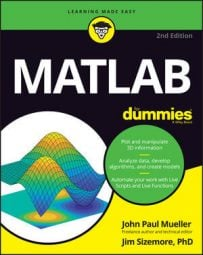##### MATLAB For DummiesYou can perform all sorts of math operations with matrices in MATLAB. You may find yourself needing to create powers of matrices or using complex numbers. MATLAB to the rescue!

## Creating powers of matrices

Sometimes you need to obtain the power or root of a matrix. The most common method is to use the circumflex (^) to separate the matrix from the power to which you want to raise it. To see how this works, type v = [1, 2; 3, 4]^2 and press Enter. The output is the original matrix squared, as shown here:

```v =
7 10
15 22```

You can obtain the same result using the mpower() function. Try it by typing w = mpower([1, 2; 3, 4], 2) and pressing Enter. You see the same output as when using the circumflex.

To obtain the root of a matrix, you use a fractional value as input. For example, to obtain the square root of the previous example, you use a value of 0.5. To see this feature in action, type x = [1, 2; 3, 4]^0.5 and press Enter. You see the following output:

```x =
0.5537 + 0.4644i 0.8070 - 0.2124i
1.2104 - 0.3186i 1.7641 + 0.1458i```

It’s even possible to obtain the inverse of a matrix by using a negative power. For example, try typing z = [1, 2; 3, 4]^(–1) and pressing Enter (notice that the –1 is enclosed in parenthesis to avoid confusion). You see the following output:

```z =
-2.0000 1.0000
1.5000 -0.5000```

MATLAB also provides the means for performing an element-by-element power or root of a matrix using the bsxfun() function and the @power handle. To see how this works, type aa = bsxfun(@power, [1, 2; 3, 4], 2) and press Enter. You see the following output, in which each element is multiplied by itself:

```aa =
1  4
9 16```

## Working element by element

You can use the bsxfun() function to perform tasks element by element. For example, to find the square of the matrix [1, 2; 3, 4], you type aa = bsxfun(@power, [1, 2; 3, 4], 2) and press Enter. Of course, the bsxfun() function provides all sorts of function handles, and you can see them all by typing help(‘bsxfun) and pressing Enter.

The problem is that the bsxfun() function requires quite a bit of typing, so you might not want to use it all the time. An alternative to using this function involves using the dot (.) operator. For example, to obtain the square of the previous matrix using the dot operator, you type ab = [1, 2; 3, 4].^2 and press Enter. The output is as you expect:

```ab =
1  4
9 16```

Notice that the dot goes between the matrix and the circumflex. You can use the dot operator in every other circumstance you can think of to modify MATLAB behavior to work element by element. For example, to perform element-by-element multiplication, you place the dot operator in front of the multiplication operator. To try the multiplication, type ac = [1, 2; 3, 4] .* [5, 6; 7, 8] and press Enter.

You see the following output:

```ac =
5 12
21 32```

## Using complex numbers

Complex numbers consist of a real part and an imaginary part. MATLAB uses the i and j constants to specify the imaginary part of the number. For example, when you compute the square root of the matrix [1, 2; 3, 4], you obtain an output that contains imaginary numbers. To see this for yourself, type ad = [1, 2; 3, 4]^0.5 and press Enter. You see the following result:

```ad =
0.5537 + 0.4644i 0.8070 - 0.2124i
1.2104 - 0.3186i 1.7641 + 0.1458i```

The first column of the first row contains a real value of 0.5537 and an imaginary value of 0.4644i. The i that appears after the value 0.4644 tells you that this is an imaginary number. The j constant means the same thing as the i constant, except that the j constant is used in electronics work.

You can perform tasks with imaginary numbers just as you would any other number. For example, you can square the ad matrix by typing ae = ad^2 and pressing Enter. The result might not be what you actually wanted, though:

```ae =
1.0000 + 0.0000i 2.0000 + 0.0000i
3.0000 - 0.0000i 4.0000 + 0.0000i```

After a matrix includes imaginary numbers, you need to convert them to obtain a desired format. For example, if you type af = int32(ad^2) and press Enter, you obtain the desired result, shown here:

```af =
1   2
3   4```

The int32() function performs the required conversion process for you. Of course, using int32(), or any other function of the same type, at the wrong time can result in data loss. For example, if you type ag = int32([1, 2; 3, 4]^0.5) and press Enter, you lose not only the imaginary part of the number but the fractional part as well. The output looks like this:

```ag =
1   1
1   2```

MATLAB assumes that you know what you’re doing, so it doesn’t stop you from making critical errors. The output conversion functions are

• double()

• single()

• int8()

• int16()

• int32()

• int64()

• uint8()

• uint16()

• uint32()

• uint64()

## Working with exponents

You use matrix exponential to perform tasks such as solving differential equations. MATLAB provides two functions for working with exponents. The first is the expm() function, which performs a standard matrix exponential. For example, when you type ah = expm([1, 2; 3, 4]) and press Enter, you see this result:

```ah =
51.9690 74.7366
112.1048 164.0738```

MATLAB also makes it easy to perform element-by-element exponential using the exp() function. To see how this works, type ai = exp([1, 2; 3, 4]) and press Enter. You see the following output:

```ai =
2.7183 7.3891
20.0855 54.5982```